The Arduino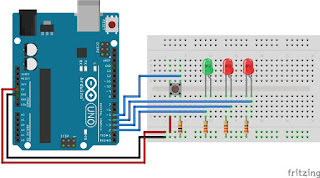is an open-source single-board micro controller, derived from the Wiring platform, designed to facilitate electronic use in a variety of fields. The hardware has an Atmel AVR processor and the software has its own programming language. Currently Arduino is very popular all over the world. Many beginners learn to get to know robotics and electronics through Arduino because it's easy to learn. But not only beginners, hobbyists or professionals are also happy to develop electronic applications using Arduino. The language used in Arduino is not a relatively difficult assembler, but a simplified C language with the help of Arduino libraries. So this time we will discuss the basics of the Arduino programming language.

Arduino structure

Every Arduino program (usually called sketch) has at least two functions (usually called routines).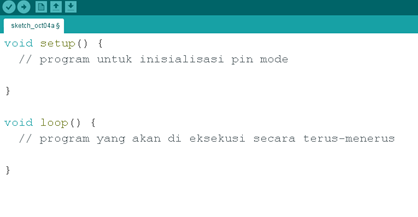All code placed between braces {} will be executed when the program starts and will stop if we remove the Arduino power supply from the power source.

1.       Syntax

One of the things that makes the C language quite troublesome is the formating elements that must be understood (however, this also makes the C language a format that must be understood (however, this also makes C a powerful programming language). If we master it then we will not be too difficult to program Arduino.

a. // (single line comment

b. / * * / (multi line comment)

c. { }(curly brackets)

d. ; (semicolon)

a.    //(single line comment

This code is used to make notes on the program. If in the program we type // then whatever is typed on the following line the // sign will not be read by the program.

Example:

void loop ()

{

// this line will not be read by the program ... the program read

}

b.   /* */(multi line comment)

If we want to make notes on the program and it has quite a lot of words then we can use / ** /. Anything that is typed between these two alerts will not be executed by the program.

Example:

void loop ()

{

/ *

whatever is typed between these marks will not be read by the program

* /

}

c.   { }(curly brackets)

Used to define the start and end of the program code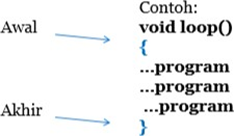d.   ;(semicolon)

Each line of code must end with a sign;

(usually this sign that beginners often forget to use so that the program cannot be compiled)

Example:

void loop () {

digitalWrite (LED, HIGH);

delay (500);

digitalWrite (LED, LOW);

delay (500);

}

2.       Variable

Variables are places to store data. Variables have names, values, and data types. The statement is usually called a declaration.

int (integer)

The most commonly used data type, it stores 2 bytes (16 bits) of data. Stores values ​​from -32,768 to 32,768.

long (long)

Used when the data is greater than integer data. It uses 4 bytes (32 bits) of RAM memory and has a range of 2,147,483,648 and 2,147, 483,648.

boolean (boolean)

Simple variable that stores True or False values. Very useful because it only uses 1 bit of RAM memory.

char (character)

Store a character using ASCII code (example: 'A' = 65). 1 byte (8 bits) RAM memory. Arduino uses strings as an array of many chars.

float (float)

Used for floating point math (decimals). The memory used is 4 bytes (32 bits) of RAM, a range of values ​​between -3.4028235E + 38 and 3.4028235E + 38.

3.      Mathematical Operations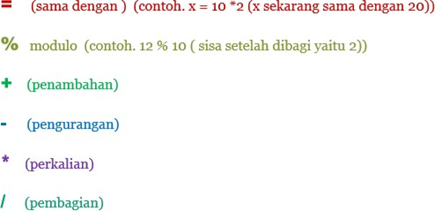Operators are used to manipulate values ​​(they work just like simple math).

4.       Comparison Operators

Operators used for logical comparisons

== (same as)

(for example, 12 == 10 F ALSE or 12 == 12 TRUE)

! = (not the same as)

(for example, 12! = 10 TRUE or 12! = 12 F ALSE)

<(less than)

(for example, 12 <10 F ALSE or 12 <12 FALSE or 12 <14 TRUE)

> (greater than)

(for example, 12> 10 TRUE or 12> 12 F ALSE or 12> 14 FALSE)

5.     Control Structure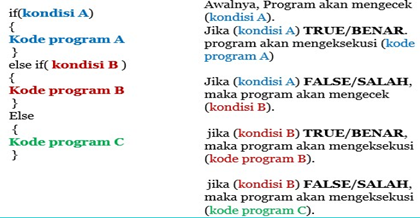for (int i = 0; i <#repeats; i ++) {}

(counting up i ++ or under i--)

Used when we want to code a value multiple times.

6.       Digital

pinMode(pin, mode);

Used to set pin mode, Pin is the pin number you want to use (pin 0 to pin 19). Mode can be INPUT or OUTPUT.

Example:

pinMode (13, INPUT);

"It means we use pin 7 on Arduino as output."

7.       Analog

Even though the Arduino is a digital machine, it can also be used to operate analog (with a few tricks).

When the input from the analog pin is set as INPUT, we can read the input voltage. The values ​​start at 0 (for 0 volts) and 1024 (for 5 volts).

int analogWrite (pin, value);

Some of the pins of the Arduino board support the PWM (pulse with modulation) pins, namely pins (3, 5, 6, 9, 10, 11). The resulting value varies between 0 (0% duty cycle of approximately 0 volts) and 255 (100% duty cycle of approximately 5 volts).

Example:

int analogWrite (6,200);

"The program will give a 200 pwm value on pin 6, so that the pin is hardware connected to the voltage (+5 volts)"

That was the basic Arduino basics that must be understood, to make it easier for you to learn the Arduino microcontroller